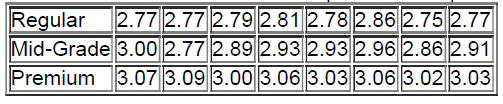×
Get Full Access to Elementary Statistics - 12 Edition - Chapter 10.3 - Problem 25bsc
Get Full Access to Elementary Statistics - 12 Edition - Chapter 10.3 - Problem 25bsc

×

# Regression and Predictions. Exercises use | Ch 10.3 - 25BSCISBN: 9780321836960 18

## Solution for problem 25BSC Chapter 10.3

Elementary Statistics | 12th Edition

• Textbook Solutions
• 2901 Step-by-step solutions solved by professors and subject experts
• Get 24/7 help from StudySoup virtual teaching assistantsElementary Statistics | 12th Edition

4 5 1 329 Reviews
23
1
Problem 25BSC

Regression and Predictions. Exercises 13–28 use the same data sets as Exercises 13–28 in Section 102. In each case, find the regression equation, letting the first variable be the predictor (x) variable. Find the indicated predicted value by following the prediction procedure summarized in Figure 10-5.

Gas Prices One gas station not included in the table below had a listed price of $2.78 for regular gas. Find the best predicted price of premium gas at this station. Is the result close to the actual price of$2.93 for premium gas?Step-by-Step Solution:

Step 1 of 1 :

Given

FigureGas Prices One gas station not included in the table below had a listed price of $2.78 for regular gas. Given data  x y 2.77 3.07 2.77 3.09 2.79 3 2.81 3.06 2.78 3.03 2.86 3.06 2.75 3.02 2.77 3.03 Now we have to determine the equation of the regression line. The table is given below.  x y xy2.77 3.07 8.5039 7.6729 2.77 3.09 8.5593 7.6729 2.79 3 8.37 7.7841 2.81 3.06 8.5986 7.8961 2.78 3.03 8.4234 7.7284 2.86 3.06 8.7516 8.1796 2.75 3.02 8.305 7.5625 2.77 3.03 8.3931 7.6729The formula of the the regression line.= a + bx whereis the dependent variable (that’s the variable that goes on the Y axis). x is the independent variable (i.e. it is plotted on the X axis). b is the slope of the line and a is the y-intercept. Where a =and b =The first step in finding a linear regression equation is to determine if there is a relationship between the two variables. a =a =a =a = 2.566 b =b =b =b = 0.1717 b0.172 The formula of the the regression line.= a + bx Substitute a = 2.566 and b = 0.172= 2.566 + 0.172 x Therefore the regression line is= 2.566 + 0.172 x . Now we need to find the best predicted price of premium gas at this station. The formula of the best predictionis=The regression line does not fit the data well, so the best predicted value is=$3.05.

The predicted price is not very close to the actual price of $2.93. Step 2 of 1 ###### Chapter 10.3, Problem 25BSC is Solved ##### Textbook: Elementary Statistics ##### Edition: 12 ##### Author: Mario F. Triola ##### ISBN: 9780321836960 Since the solution to 25BSC from 10.3 chapter was answered, more than 459 students have viewed the full step-by-step answer. This textbook survival guide was created for the textbook: Elementary Statistics, edition: 12. This full solution covers the following key subjects: gas, Find, price, predicted, premium. This expansive textbook survival guide covers 121 chapters, and 3629 solutions. The full step-by-step solution to problem: 25BSC from chapter: 10.3 was answered by , our top Statistics solution expert on 03/15/17, 10:30PM. Elementary Statistics was written by and is associated to the ISBN: 9780321836960. The answer to “?Regression and Predictions. Exercises 13–28 use the same data sets as Exercises 13–28 in Section 102. In each case, find the regression equation, letting the first variable be the predictor (x) variable. Find the indicated predicted value by following the prediction procedure summarized in Figure 10-5.Gas Prices One gas station not included in the table below had a listed price of$2.78 for regular gas. Find the best predicted price of premium gas at this station. Is the result close to the actual price of \$2.93 for premium gas?” is broken down into a number of easy to follow steps, and 89 words.

Unlock Textbook Solution